research papersJOURNAL OFSYNCHROTRONRADIATION
ISSN: 1600-5775
Volume 18| Part 3| May 2011| Pages 515-521

Geometrical layout and optics modelling of the surface science beamline station at the SESAME synchrotron radiation facility

aSynchrotron Light for Experimental Science and Application in the Middle East (SESAME), PO Box 7, Allan 19252, Jordan, bPhysics Department, The Hashemite University, Zarqa 13115, Jordan, and cEuropean Synchrotron Radiation Facility, BP 220, 38043 Grenoble Cedex, France
*Correspondence e-mail: wael.salah@sesame.org.jo

(Received 7 September 2010; accepted 7 March 2011; online 19 March 2011)

The layout and the optical performance of the SGM branch of the D09 bending-magnet beamline, under construction at SESAME, are presented. The beamline is based on the Dragon-type design and delivers photons over the spectral range 15–250 eV. One fixed entrance slit and a movable exit slit are used. The performance of the beamline has been characterized by calculating the mirror reflectivities and the grating efficiencies. The flux and resolution were calculated by ray-tracing using SHADOW. The grating diffraction efficiencies were calculated using the GRADIF code. The results and the overall shapes of the predicted curves are in reasonable agreement with those obtained using an analytical formula.

1. Introduction

SESAME (Synchrotron light for Experimental Science and Application in the Middle East) is a third-generation light source under construction, which will provide the Middle East region with synchrotron radiation. SESAME aims to foster outstanding science and technology in the Middle East and neighbouring countries. It will build scientific and technical capacity and facilitate collaboration between the region's scientists and engineers. Moreover, SESAME will foster closer links between peoples with different traditions, political systems and beliefs, in a region where better understanding is much needed.

One of the bending-magnet source beamlines that SESAME intends to complete within the next year is dedicated to soft X-ray vacuum-ultraviolet (XVUV) and absorption fine structure (XAFS). This beamline (denoted D09) will provide photons in the range 15 eV–10 keV in two independent branches. The beam will be split using a beam splitter. The first branch (XVUV), which is described here, is designed to supply radiation in the photon energy range 15–250 eV. This energy range allows excitation or ionization processes from molecular valance shells as well as covering the region in which double and triple ionization phenomena play a prominent role. The second branch (XAFS) will cover the high-energy range 640 eV–10 keV, needed for X-ray absorption spectroscopy of adsorbed species with atomic number greater than Z = 9.

Up to now, over 30 VUV beamlines have been built at the synchrotron radiation facilities in the world. However, there are few high-flux and high-resolution VUV beamlines in the photon energy range less than 30.0 eV on medium-energy rings.

The aim of this paper is to educate beamline scientists about the decisions involved in specifying the beamline components of the XVUV branches of D09, and to project the capabilities that future users can expect on the beamline. Experienced scientists will be encouraged to remain in, or return to, their home' region to pursue their research interests at SESAME, and graduate students and young researchers will no longer have to go abroad for advanced training. This will make SESAME a unique multi-disciplinary centre in the region.

In this paper we describe the geometrical layout and evaluate the performance of the 15–250 eV branch line. The beamline performance is simulated by ray tracing using the SHADOW (Cerrina & Sanchez del Rio, 2009) package under XOP (Sanchez del Rio & Dejus, 2004). Grating efficiencies are calculated using the GRADIF code (Neviere et al., 1974, 1988).

2. Beamline description

2.1. Source

The X-ray source is a dipole bending magnet of radius 5.73 m, operating at 1.455 T. The source size is 231.1 µm and 79.5 µm r.m.s. for the horizontal and vertical plane, respectively. The electron beam energy is 2.5 GeV with horizontal and vertical divergences (r.m.s.) of 267.1 µrad and 13.3 µrad, respectively (Salah et al., 2009). The intensity of the storage ring is 400 mA and the beamline collects 6 mrad horizontally from the bending-magnet radiation. Using these parameters the source was generated using SHADOW, as shown recently by Salah et al. (2009) using a bending-magnet source.

2.2. Optical design

The D09 beamline X-ray optics is shown in Fig. 1. At the front-end a bent cylindrical horizontally focusing mirror (HFM; focal distances p1 = 9.67 m, p2 = 15.076 m, magnification 1.56, radius of curvature 10408 cm) is located 9.67 m downstream from the source. It collects 6 mrad of the synchrotron radiation, deflects the beam sideways, and focuses in the horizontal direction at the monochromator exit slit. This Glidcop mirror is platinum-coated with an optically active surface area of 570 × 42 mm. The surface of the mirror is polished to a r.m.s. roughness of 1.0 nm and to a r.m.s. residual slope error of 10 arcsec. The angle of incident is 83.5° and the reflectivity is shown in Fig. 2. The HFM acts as a power filter by absorbing most of the incoming thermal load; it protects the downstream optical elements from distortion due to thermal loading. The second optical element is a vertically focusing mirror (VFM; radius of curvature 9063 cm) which is located 12.67 m downstream from the source. The mirror deflects the photon beam vertically onto the entrance slit of the monochromator (focal distances p1 = 12.67 m, p2 = 4.21 m, demagnification 3.01). It is also platinum-coated on Glidcop with an optically active surface area of 400 × 70 mm. The surface of the mirror is polished to a r.m.s. roughness of 0.5 nm and to a r.m.s. residual slope error of 0.5 arcsec. The angle of incidence is 86.0°. The VFM is followed by a spherical-grating monochromator (SGM) placed at 19.7 m from the source. It follows the Dragon concept (Chen, 1987). This monochromator is the main optical element, whose task is to diffract and focus the photon beam vertically at the exit slit. The SGM has been chosen owing to its simple wavelength-scanning mechanism and its high performance. The monochromator has been designed to work in the first outside order with a fixed included angle of 164° and has three interchangeable diffraction gratings. The low-energy grating with a ruling density of 204 lines mm−1 covers the photon energy range from 15 to 45 eV, the medium-energy grating with a ruling density of 597 lines mm−1 covers the energy range from 45 to 130 eV, and the high-energy grating with a ruling density of 1500 lines mm−1 covers 130 to 220 eV. Each grating has an optically active area of 150 mm (perpendicular to the groove direction) × 35 mm (parallel to the groove direction). The gratings can be interchanged under vacuum by a lateral translation across the beam, and each grating has independent roll and yaw adjustment. The rotation about the pole of the grating parallel to the grooves (to change the energy of the diffracted radiation) is accomplished through a standard sine-bar mechanism. The entrance slit is fixed at 16.882 m downstream from the source. The entrance slit opening is variable over 0.005–3.0 mm to allow a suitable compromise between intensity and resolution. The exit slit translates from its mean position (2429 cm downstream from the source) along the beam path to remain in the focus of the grating as it is rotated to select the appropriate wavelength or photon energy. It has a variable opening from 0.005 to 3.0 mm. The translation distance from the mean position of the exit-slit length ranges from 24.3 cm along the optical axis. This movement allows the contribution to the overall resolution from the grating defocus aberration to be suppressed, as explained later. To refocus the beam from the exit slit to the sample, a gold-coated ellipsoidal mirror is placed at 26.83 m downstream from the source to refocus the beam both horizontally and vertically on the sample position at 2700 cm from the source. The mirror r.m.s. surface roughness is 0.5 nm. The figure slope accuracy is 2.0 arcsec. The ellipsoid has an approximated major radius (optical, tangential) of 474.8 cm and minor radius (sagittal) of 2.31 cm. It deflects the photon beam by 8.0° with a demagnification of 14.3. The geometrical parameters and optical specification of all the optical elements are summarized in Table 1.

 Table 1Beamline parameters
 Bending-magnet dipole Size 231.1 × 79.5 µm Divergence 267.1 × 13.3 µrad HFM Distance from source 9.67 m Horizontal beam deflection 13° Cylindrical radius 104.80 m (optimized value) Material Pt on Glidcop, water-cooled Active optical area 570 × 42 mm Slope error 1.0 arcsec Microroughness 1.0 nm r.m.s VFM Distance from HFM 3 m Vertical beam deflection 8 Cylindrical radius 90.63 m Material Pt on Glidcop Active optical area 400 × 70 mm Slope error 0.5 arcsec Microroughness 0.5 nm r.m.s Demagnification 3 Entrance slit Distance from VFM 4.212 m Traverse ±50 mm Slit width 0.005–3.00 mm Slit length 35 mm Spherical-grating monochromator Distance from entrance slit 2.818 m Included angle 164° Spherical radius 26.19 m Grating material Au on fused silica Active optical area 35 × 150 mm Diffraction order Positive 1 Maximum rotation from zero order 4° Rotation step accuracy 0.5 arcsec Gratings energy range 15–45, 45–130, 130–220 eV Groove density 204, 597, 1500 lines mm−1 Laminar groove depth 60, 23.5, 16 nm Laminar groove width 2549, 1005, 480 nm Microroughness 0.5 nm r.m.s Slope error <1 arcsec Translatable exit slit Distance from grating 4.59 m Traverse 300 mm Slit width 0.005–3.00 mm Slit length 35 mm Refocusing ellipsoidal mirror Distance from exit slit 2.546 m Beam deflection 8° Semi-major axis 1.773 m Semi-minor axis 0.1113 m Active optical area 300 × 40 mm Material Pt on Glidcop Distance from sample 1.0 m Slope error 2 arcsec Microroughness 0.5 nm r.m.s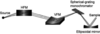Figure 1 D09 beamline optical components layout.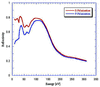Figure 2 Reflectivity of the Pt-coated HFM for the σ- and π-polarization as a function of incident photon energy (in eV) at the mirror surface. The incident angle is 83.5° and roughness r.m.s. is 1.0 arcsec.

3. Performance calculations and ray tracing

3.1. Mirror optics

The reflectivity of the mirror depends on the grazing angle, photon energy and mirror coating. The mirror's performance is limited by the surface errors which are classified by waviness, or slope error, and roughness (Dagnal, 1986). Slope errors are usually specified as the angular root-mean-square (r.m.s.) variation from the ideal figure along the mirror and perpendicular to its length. These errors arise from long-period ripples on the mirror surface. Waviness or slope errors degrade the focusing performance of the mirror because they deflect the photons from their ideal path. They can be treated using a geometric optics model (Sanchez del Rio & Marcelli, 1992). Roughness is usually specified as the r.m.s. of the surface height. Roughness is caused by atomic-scale surface height fluctuations. Surface roughness affects the reflectivity and smooths out the intensity step at the critical energy. Surface roughness also causes a diffuse scattering background. More precisely, roughness produces diffraction of the photon beam (incoherent reflection) (Dagnal, 1986). SHADOW has an option for including roughness by Monte Carlo sampling the diffracted rays (Singh et al., 1996). Details of the surface error contribution in ray tracing can be found by Salah et al. (2009), and we used here the same framework. The mirror reflectivity for a smooth perfect surface is given by Fresnel formulae (Born & Wolf, 1989). The reflectivity of the rough surface is calculated from that of the smooth one by the Debye–Waller exponential factor (Salah et al., 2009),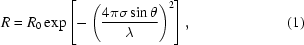where R0 is the ideal surface reflectivity calculated by the Fresnel equation, σ is the r.m.s. surface roughness, θ is the grazing incident angle, and λ is the photon wavelength. The reflectivity of the HFM as a function of photon energy is obtained by means of the XOP code (Sanchez del Rio & Dejus, 2004) as illustrated in Fig. 2. The same procedure is followed for the analysis of the other mirrors.

A fundamental role of the first mirror is to remove much of the beam power and manage the heat load using a suitable cooling system. It receives 230.5 W from the source, as calculated by XOP. A small fraction of this power, which will be used by the beamline, is specularly reflected in the reflected beam. It corresponds to wavelengths longer than the critical wavelength, and totals ∼3 W. The rest of the beam power (227.5 W) enters the mirror and is mostly absorbed. There is, however, a fraction of it that is re-scattered owing mainly to inelastic processes. It is important to estimate how much of the power is re-radiated for two reasons: one is to know exactly the incoming power in order to design the cooling system, and to be able to compute the thermal deformation of the mirror by finite-elements analysis; the second is that the re-scattered radiation heats the electromechanics in the mirror chamber, producing instabilities. Therefore, it is important to accurately calculate the re-radiated power. For this we have implemented a Monte Carlo particle-tracking code using the PENELOPE package (Salvat et al., 2008). The results are shown in Fig. 3. These results show that from the 227.5 W that enter the mirror, simulated as a thick copper block, 215.1 ± 0.6 W are absorbed and 12.4 ± 0.2 W are re-scattered upwards from the mirror.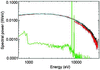Figure 3 Source spectral power as a function of the photon energy (blue, 231 W), source spectrum without power specularly reflected (black, 227.5 W), energy spectrum of the absorbed power in the mirror (red, 215.1 W, 94.5%) and spectrum of the power scattered by the first mirror (green, 12.4 W, 5.5%). The Compton profiles and the Cu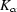and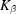lines can be observed.

3.2. Diffraction grating optics

The diffraction grating is the heart of most soft X-ray spectroscopy beamlines (Welford, 1965; Hunter, 1985). The monochromator used here works for a constant included angle αβ = 2θ = 164°, where α and β are the incident and diffracted angles, respectively, and θ is the constant included angle. Therefore the grating equation becomes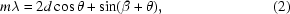where λ is the radiation wavelength, d is the groove spacing, and m is an integer called the diffraction or spectral order.

The grating efficiency has been the subject of intense studies during recent years (Bowler et al., 2001; Paerels et al., 1998; Reininger et al., 2004) with computer codes dedicated to the calculation of the grating efficiency. Among these codes we find the GRADIF code of Neviere et al. (Neviere et al. 1974; Neviere & Monteil, 1996) and the EFFEM2 code of Kim & Oh (2001). GRADIF solves the differential equation from electromagnetic theory by integrating the equation through the grating. EFFEM2 uses a differential method like GRADIF with an extension for composing the Jones matrix in order to show the polarization behaviour.

The diffraction efficiency of a transmission grating is defined as the ratio of the intensity observed in a spectral order at a given wavelength normalized to the intensity without the grating in the beam.

According to Kirchhoff's integral and the Fraunhofer diffraction theory (Born & Wolf, 1989) and the basic theory of gratings (Haber, 1950; Noda et al., 1974), the intensity distribution is approximated in the Fraunhofer limit by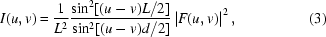with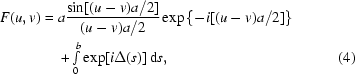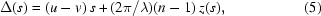where L is the grating total width, a is the slit width, b is the bar width, u =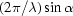and v =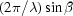, s is the dimension in the plane of the grating perpendicular to the bars, Δ(s) is the phase-shift contribution of the bars, and z(s) is the geometrical cross section of the bars as a function of s along the direction of the incoming radiation. The first term in the expression of I(u, v) describes the interference between the large number N = L/d of slits in the grating. The two terms in F(u, v) describe the slit and bar contribution to the diffraction patter, respectively. Using the results from Schnopper et al. (1977) and Eidmann et al. (1990) and the condition for constructive interference,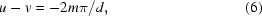the integral term in (4)can be written as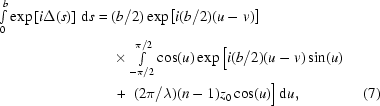where z0 is the bar thickness, n = n1+in2 is the complex refraction index of the grating material (Henke et al., 1982), and a/d is the ratio of the gap between the grating bars a and the bar period d.

The exit-slit position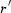is obtained from the focusing equation in the diffraction plane,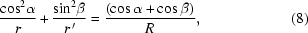where r andare the entrance- and exit-slit position and R is the radius of the spherical grating.

The resolving power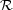of a grating is a measure of its ability to separate adjacent spectral lines of average wavelength λ. It is usually expressed as the dimensionless quantity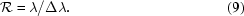Here, Δλ is the limit of resolution, the difference in wavelength between two lines of equal intensity that can be distinguished. To determine the performance of the monochromator, we need to determine the appropriate width values for the entrance and exit slits. These slits are located at the image planes of the first and second optical element. The entrance slit should be set to an opening that brackets the source image. Ideally, the optical system must be designed to relay and image the source consistent with the required resolution. In many instances the diffracted power depends on the polarization of the incident light. P-plane or TE-polarized light is polarized parallel to the grating grooves, while S-plane or TM-polarized light is polarized perpendicular to the grating grooves. These states of polarization are not covered by the approximation in equation (3). However, the GRADIF code takes into account the polarization state in the grating efficiency calculation. As an example of GRADIF output, Figs. 4and 5show the first-order theoretical total-, S- and P-efficiency as a function of the radiation photon energy for the third and the three gratings, respectively. The total efficiency is the average of the S- and P-efficiency whose curve will be exactly between the P- and S-efficiency curves. The peak in efficiency, as shown in these figures, occurs at the blaze wavelength. Moreover, the efficiency curves shown in Fig. 5are smooth for the second and third grating whereas anomalies that exist at low frequencies are shown in the curve of the first grating. Anomalies are locations at which the efficiency changes abruptly. Anomalies observed in the S-polarization curve are called Rayleigh anomalies and the anomalies observed in the P-polarization are called resonance anomalies (Hessel & Oliner, 1965). For comparison with simple analytical models, the efficiency of the grating is also calculated by equation (3). The grating efficiency results obtained from (3)are compared with those obtained using the GRADIF code as shown in Fig. 4. This comparison shows that the two results are in reasonable agreement within ∼10%.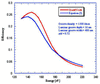Figure 4 First-order theoretical efficiency as a function of the radiation photon energy for the third grating.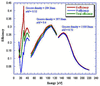Figure 5 First-order theoretical S-, P- and total-efficiency of the three laminar grating as a function of the radiation photon energy.

From the calculations described above we conclude that the grating efficiency is extremely sensitive to the wavelength and grating parameters. Moreover, the gratings are more efficient at the highest energies.

The grating instrumental line profile is the apparent spectral broadening produced by the grating when illuminated with a purely monochromatic wavelength. The line profile has finite width and is known as the instrumental line profile' or `instrumental band-pass'. The instrumental profile can be related to the energy band-pass. SHADOW can be used to simulate the instrumental line profile versus wavelength by setting a monochromatic source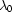= 70.85 Å (E = 175 eV) and then calculating the integrated intensity into the exit slit as a function of grating positions that correspond to wavelengths around. The results of these simulations are shown in Fig. 6for several entrance- and exit-slit widths set equal and varied from 500 to 700 µm. These are the spectra expected with a strictly monochromatic source and with the gratings rotated to match the wavelength in the abscissa.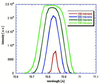Figure 6 Instrumental line function: plot of the intensity (arbitrary units) obtained when rotating the gratings when a monochromatic source= 70.85 Å (175 eV) is used. The different curves are for different values of the entrance and exit slit.

The linear dispersion of the monochromator depends on the groove density. Closer grating lines will result in a large separation in z of two lines in wavelength defining a continuum interval. In order to analyze the grating dispersion we simulated a continuum source around= 70.85 Å. The grating dispersion is analyzed by plotting the z position in the exit-slit plane versus the wavelength. The results are obtained using SHADOW and schematized in Fig. 7, where the entrance- and exit-slit widths are set to 700 µm and the groove density is 1500 lines mm−1. The resolution corresponding to the closed exit slits condition (no intensity) is defined by the horizontal line (at z = 0) as shown at the top of the figure. The thickness and the tail observed in this figure are due to the instrumental line profile. The bottom of the figure represents the same plot at the top with a fitting with a straight line. By fitting these points we obtain the pendent p = 0.380. The pendent is used to calculate the distance to the origin of the exit slit where a line will be placed as follows,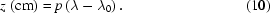This equation gives the linear dispersion which is |Δz/Δλ|. The increased band-pass owing to the exit-slit width is just the product of the reciprocal linear dispersion and the slit width. The resolving power is calculated in the limit of exit slit Δz = 0 which corresponds to a horizontal line at z = 0, and equals 698 for an entrance slit of 700 µm and 2580 for 100 µm.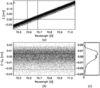Figure 7 The wavelength dispersion of the monochromator. (a) The scattered plot of coordinate z in the exit slit versus wavelength (in Å) showing the effect of the grating in dispersing different wavelengths along z. The entrance slit width is set to 700 µm with groove density = 1500 lines mm−1. The horizontal line in z = 0 defines the best resolution (closed slit),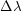= 0.101 Å, and gives a resolving power of 698.4. (b) The same plot as in (a) but with the fitted straight line removed. (c) The histogram shows the instrumental line profile. From the fitting of these points we obtain the pendent p = 0.38. The latter is used to calculate the distance to the origin of the exit slit where a line will be placed.

Since the resolving power depends on the photon energy and slit aperture, we calculate the resolving power at different photon energies and apertures. The results are shown in Fig. 8.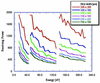Figure 8 Resolving power of the three gratings as a function of photon energy with different slit opening settings.

4. Beam focusing at the sample position and flux calculation

The SHADOW ray-tracing results, showing the ray distribution at the sample focus at a photon energy of 175.0 eV, are shown in Fig. 9.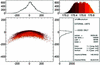Figure 9 Ray-trace simulation of the focused beam at the sample position. The focused photon beam dimension on the vertical plane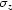(FWHM) = 48.0 ± 4 µm and on the horizontal plane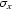(FWHM) = 158.7 ± 17 µm. The coloured histogram shows the energy resolution (entrance and exit slit 0.7 mm) giving 0.44 eV FWHM (0.18 Å).

The flux (photons s−1) at the sample position is given by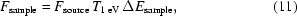where Fsample denotes the X-ray flux at the sample position, Fsource denotes the X-ray flux from the source in a 1 eV bandwidth, T1 eV denotes the system coefficient of transmission in 1 eV bandwidth, and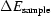is the energy bandwidth of the sample.

The coefficient T1 eV is calculated as follows,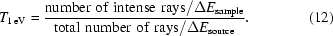The extracted results of the ray tracing are shown in Table 2. Since the flux at the sample position depends on the photon energy and slit aperture, we calculate the flux at the sample position at different photon energies and apertures using XOP as shown in Fig. 10. The total flux coming from the source, Fsource, is calculated using XOP. The obtained value is tabulated in Table 2.

 Table 2Ray-tracing focusing results
 Photon energy 175 eV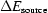4.7 eV0.43 eV Fsource 8.97 × 1013 photons s−1 (0.1% bandwidth)−1 T1 eV 0.16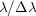404 I(u, v) 0.12 Fsample 4.2 × 1012 photons s−1 Horizontal focus spot158.7 ± 17 µm (FWHM) Vertical focus spot48.0 ± 4 µm (FWHM)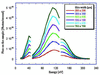Figure 10 Photon flux of the three gratings on the sample as a function of photon energy with different slit opening settings.

The SHADOW flux values should be corrected for the grating efficiency I(u, v) which is not included in the ray tracing. By multiplying the numerical result of Fsample by the efficiency we obtain the total number of photons per second integrated over all the energy bandwidth at the sample position. The obtained numerical result is also tabulated in Table 2.

5. Conclusion

We have presented a quantitative analysis and computer modelling of the surface science beamline station D09 at the SESAME synchrotron radiation facility. The performance of the beamline has been characterized by calculating the total photon intensity delivered by each of the three gratings using 500–700 µm entrance- and exit-slit widths. The grating efficiency, grating energy resolution and instrumental line profile have also been investigated. Numerical results of the grating efficiency show that the grating efficiency is extremely sensitive to the wavelengths and grating parameters.

Several hundred scientists are expected to use this multi-purpose beamline in order to carry out measurements on either angle-resolved and angle-integrated photoemission or photoelectron diffraction and dichroism studies.

Acknowledgements

We thank Eleonora Secco for power calculation using the PENELOPE Monte Carlo package.

ReferencesJOURNAL OFSYNCHROTRONRADIATION